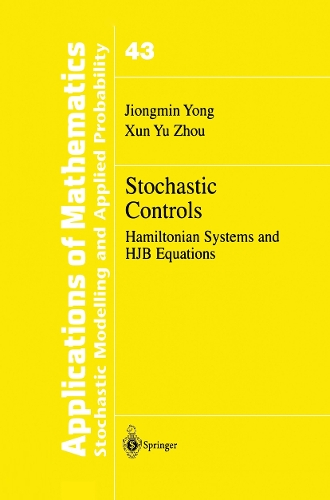•Stochastic Controls: Hamiltonian Systems and HJB Equations - Stochastic Modelling and Applied Probability 43 (Paperback)

(author), (author)
£99.99
Paperback 439 Pages / Published: 27/09/2012
• We can order this

Usually dispatched within 3 weeks

As is well known, Pontryagin's maximum principle and Bellman's dynamic programming are the two principal and most commonly used approaches in solving stochastic optimal control problems. * An interesting phenomenon one can observe from the literature is that these two approaches have been developed separately and independently. Since both methods are used to investigate the same problems, a natural question one will ask is the fol- lowing: (Q) What is the relationship betwccn the maximum principlc and dy- namic programming in stochastic optimal controls? There did exist some researches (prior to the 1980s) on the relationship between these two. Nevertheless, the results usually werestated in heuristic terms and proved under rather restrictive assumptions, which were not satisfied in most cases. In the statement of a Pontryagin-type maximum principle there is an adjoint equation, which is an ordinary differential equation (ODE) in the (finite-dimensional) deterministic case and a stochastic differential equation (SDE) in the stochastic case. The system consisting of the adjoint equa- tion, the original state equation, and the maximum condition is referred to as an (extended) Hamiltonian system. On the other hand, in Bellman's dynamic programming, there is a partial differential equation (PDE), of first order in the (finite-dimensional) deterministic case and of second or- der in the stochastic case. This is known as a Hamilton-Jacobi-Bellman (HJB) equation.

Publisher: Springer-Verlag New York Inc.
ISBN: 9781461271543
Number of pages: 439
Weight: 706 g
Dimensions: 235 x 155 x 24 mm
Edition: Softcover reprint of the original 1st ed. 199

MEDIA REVIEWS

From the reviews:

SIAM REVIEW

"The presentation of this book is systematic and self-contained...Summing up, this book is a very good addition to the control literature, with original features not found in other reference books. Certain parts could be used as basic material for a graduate (or postgraduate) course...This book is highly recommended to anyone who wishes to study the relationship between Pontryagin's maximum principle and Bellman's dynamic programming principle applied to diffusion processes."

MATHEMATICS REVIEW

This is an authoratative book which should be of interest to researchers in stochastic control, mathematical finance, probability theory, and applied mathematics. Material out of this book could also be used in graduate courses on stochastic control and dynamic optimization in mathematics, engineering, and finance curricula. Tamer Basar, Math. Review